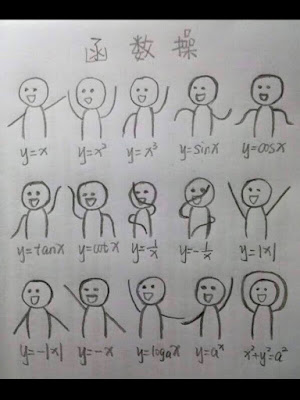## Friday, March 27, 2020

### Turn Complex Data into Numbers

Turn data into features. Turn data into feature vectors. Machine Learning models can only take numeric data. All input data must be represented numerically. For example, words need to be converted to word embeddings in some Natural Language Processing tasks.

### Training vs Inference Models

There are two major tasks in machine learning 1. build and train a model 2. deploy a model for inference. Part 1 takes known data, uses it to tune parameters of the model such as weights. Part 2 takes in unknown data, real world data or test data and calls a dot predict method on the new data.

### Normalization, Scaling Data

Normalize data, need to scale data to bound it. For example in Machine Learning, an error term can be arbitrarily large because the model can be arbitrarily bad, causing the lower bound of error term for f(x) = wx+b to be essentially unbounded. Bounding the error term by scaling the features numeric value can make the result easier to compute and make the search space easier for gradient descent.

### Bias Variance Tradeoff

High bias may refer to underfitting, where the model is too simple, not complex enough to make accurate predictions. It can also mean when the model is practically ignoring the data.

High variance may refer to overfitting. That's when the model overfits, hence cannot generate to future data well.

#### 1 comment:

1.This is a great article and your blog is also very interesting. Thank you.Street View Online

### Data analysis

Data visualization of common math formula.